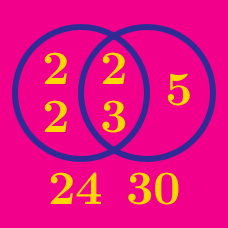Number Theory

Lowest Common Multiple

What is the least common multiple of $2$ and $3$?

What is the lowest common multiple of 39 and 65?

A bus stop has two bus lines: the red line and the blue line. The red line stops every $18$ minutes, and the blue line stops every $27$ minutes. If the buses of both lines have just simultaneously stopped at the bus stop, how many minutes later will the buses of the two lines meet at this bus stop again?

If the lowest common multiple of $3^a 5^b$ and $3^4$ is $3^5$, what is $3^a 5^b ?$

What is the least common multiple of 12 and 21?

×